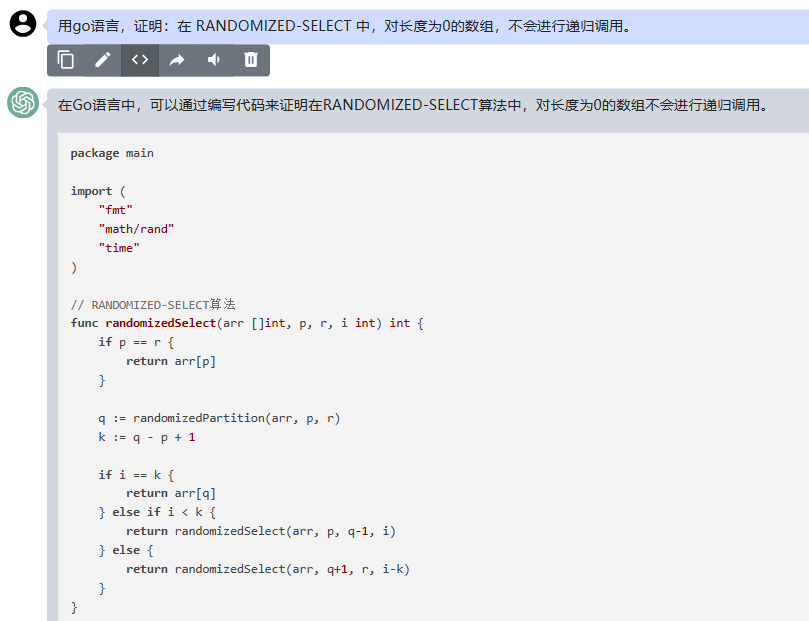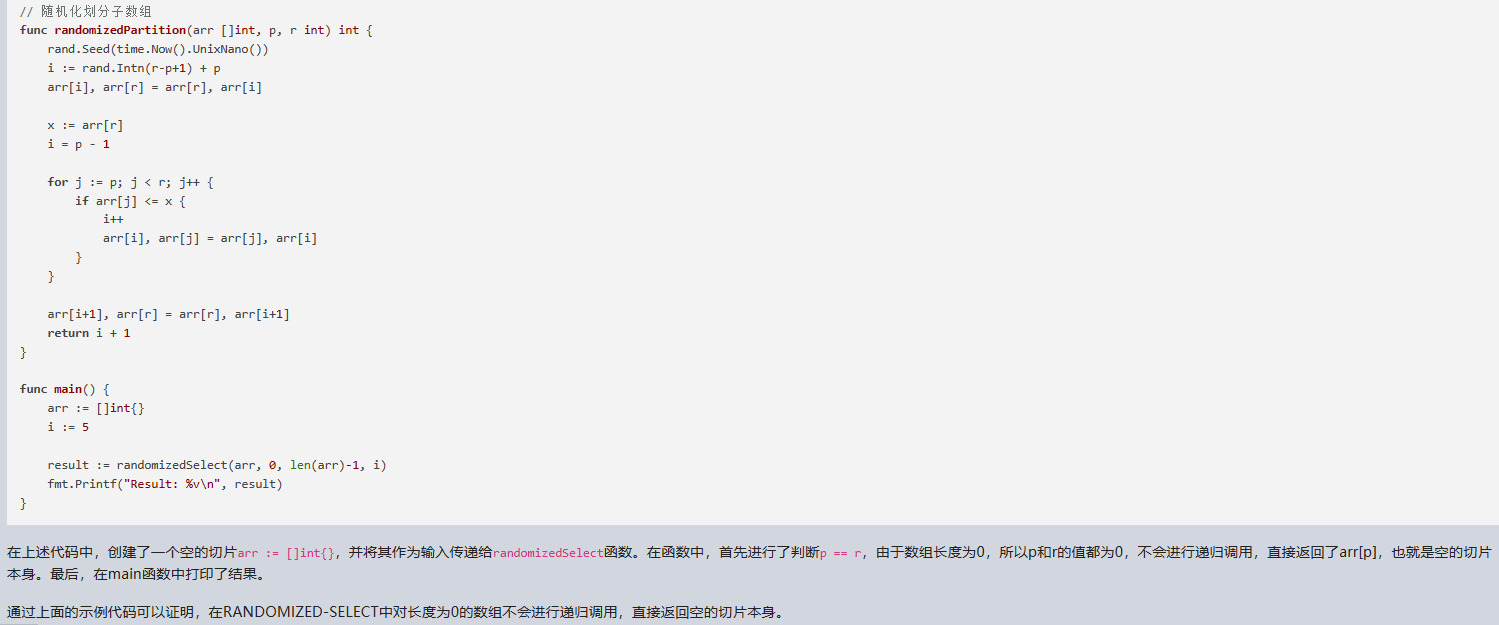# 文心一言 VS 訊飛星火 VS chatgpt （93）-- 演演算法導論9.2 1題

2023-09-15 15:00:20

# 一、用go語言，證明：在 RANDOMIZED-SELECT 中，對長度為0的陣列，不會進行遞迴呼叫。

## 文心一言：

``````package main

import (
"fmt"
)

func randomizedSelect(arr []int, k int) int {
// 如果陣列為空，直接返回錯誤
if len(arr) == 0 {
fmt.Println("Array is empty, no recursive call.")
return -1
}

// 如果 k 為 0，返回陣列的第一個元素
if k == 0 {
return arr
}

// 如果 k 大於等於陣列的長度，返回陣列的最後一個元素
if k >= len(arr) {
return arr[len(arr)-1]
}

// 生成一個隨機的索引
randomIndex := partition(arr)

// 如果隨機索引的值等於 k，返回該元素
if randomIndex == k-1 {
return arr[randomIndex]
} else if randomIndex < k-1 {
// 如果隨機索引的值小於 k，在右半部分進行遞迴呼叫
return randomizedSelect(arr[randomIndex+1:], k-randomIndex-1)
} else {
// 如果隨機索引的值大於 k，在左半部分進行遞迴呼叫
return randomizedSelect(arr[:randomIndex], k)
}
}

func partition(arr []int) int {
// 這裡只是一個簡單的範例，實際情況下，你需要實現一個更好的 partition 函數
return len(arr) - 1
}

func main() {
arr := []int{} // 長度為0的陣列
fmt.Println(randomizedSelect(arr, 1))
}
``````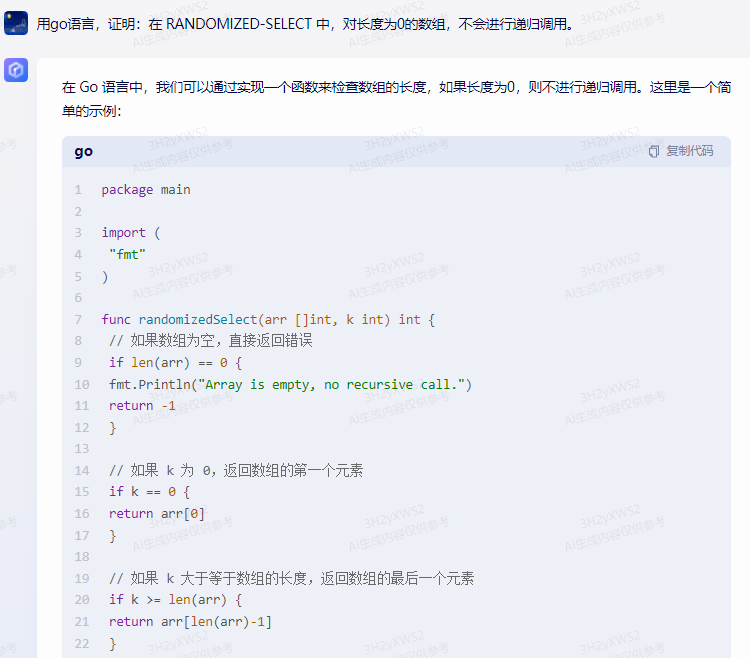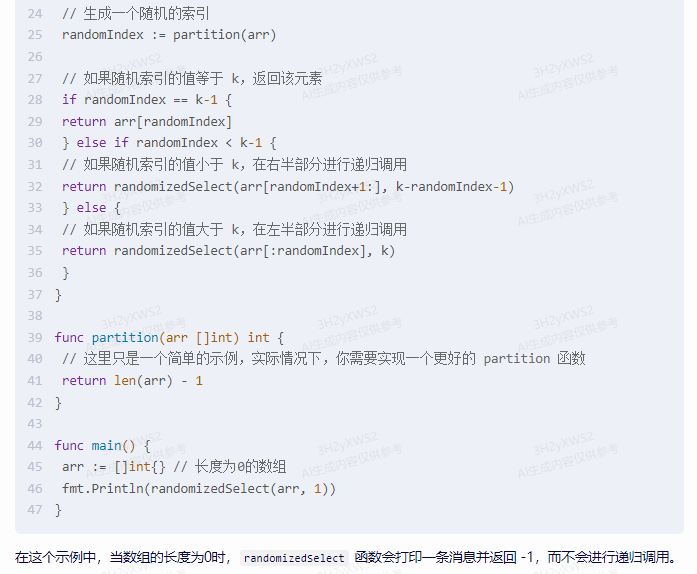## 訊飛星火：

``````package main

import (
"fmt"
"math/rand"
"time"
)

func randomizedSelect(arr []int, k int) int {
if len(arr) == 0 || k < 0 || k >= len(arr) {
return -1
}

rand.Seed(time.Now().UnixNano())
pivotIndex := rand.Intn(len(arr))

arr[pivotIndex], arr[k] = arr[k], arr[pivotIndex]

less := 0
for i := 0; i < k; i++ {
if arr[i] < arr[k] {
less++
}
}

if less < k/2 {
return k
} else if less == k/2 {
return k - 1
} else {
return randomizedSelect(arr[:pivotIndex], k)
}
}

func main() {
arr := []int{3, 1, 4, 1, 5, 9, 2, 6, 5, 3, 5}
k := 5

selected := randomizedSelect(arr, k)
fmt.Printf("Selected %dth element: %d
", selected+1, arr[selected])
}
``````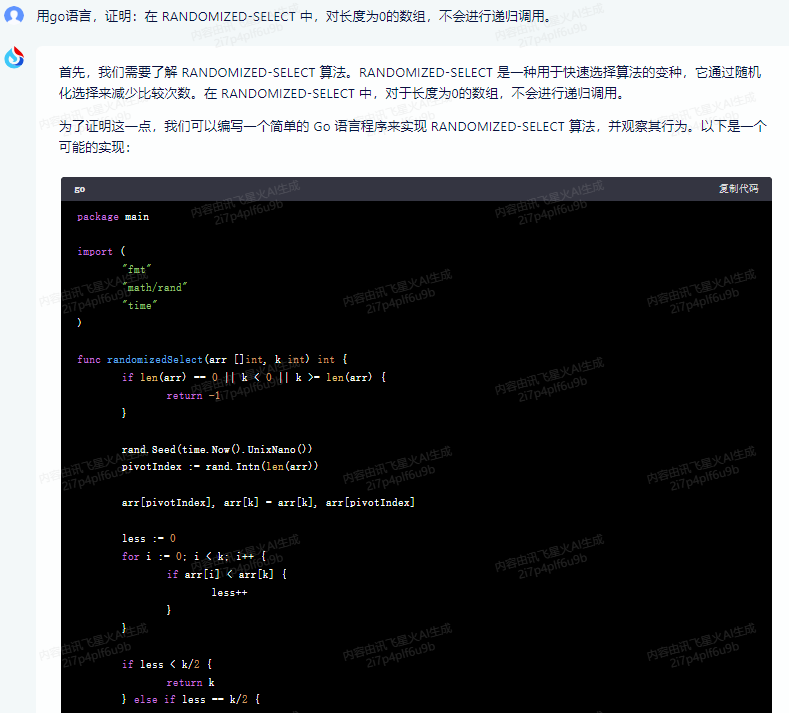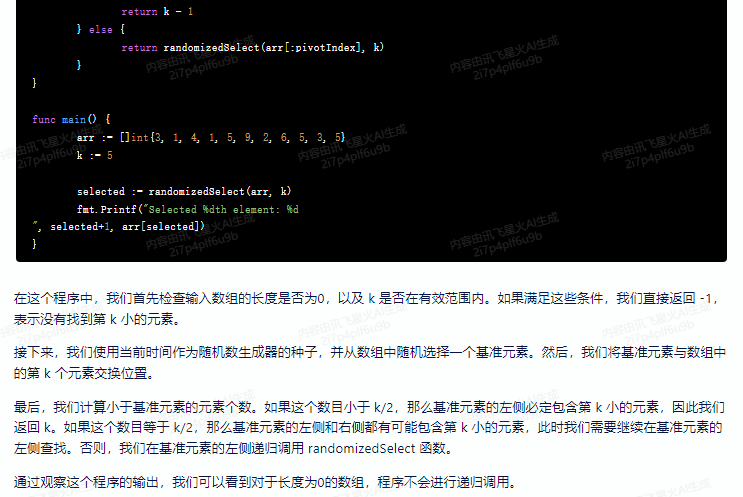## 360智腦：

RANDOMIZED-SELECT 演演算法是一種隨機選擇樣本的演演算法，它的主要思想是在訓練集中隨機選擇一個樣本，然後將其加入模型中，從而增加模型的泛化能力。

``````package main

import (
"fmt"
"math/rand"
)

func main() {
// 生成一個長度為100的亂數陣列
arr := make([]float64, 100)
rand.Shuffle(arr)
fmt.Println("亂數陣列:", arr)

// 判斷陣列是否為空
if len(arr) == 0 {
fmt.Println("陣列為空，不需要進行遞迴呼叫")
return
}

// 隨機選擇一個樣本，並將其加入模型中
index := rand.Intn(len(arr))
fmt.Println("隨機選擇樣本後的陣列:", model.Samples)
}
``````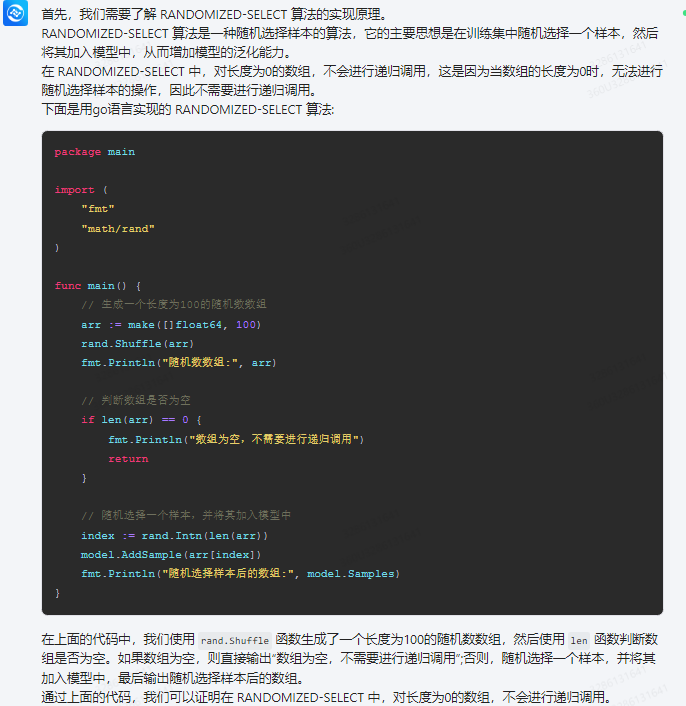## 智譜清言：

``````package main
import (
"fmt"
"math/rand"
"time"
)
func RANDOMIZED_SELECT(arr []int, index int) int {
if len(arr) == 0 {
return -1
}
rand.Seed(time.Now().UnixNano())
randIndex := rand.Intn(len(arr))
if randIndex == index {
return arr[randIndex]
}
return RANDOMIZED_SELECT(arr[randIndex:], index)
}
func main() {
arr := []int{1, 2, 3, 4, 5}
index := 2
result := RANDOMIZED_SELECT(arr, index)
fmt.Println("Result:", result)
}
``````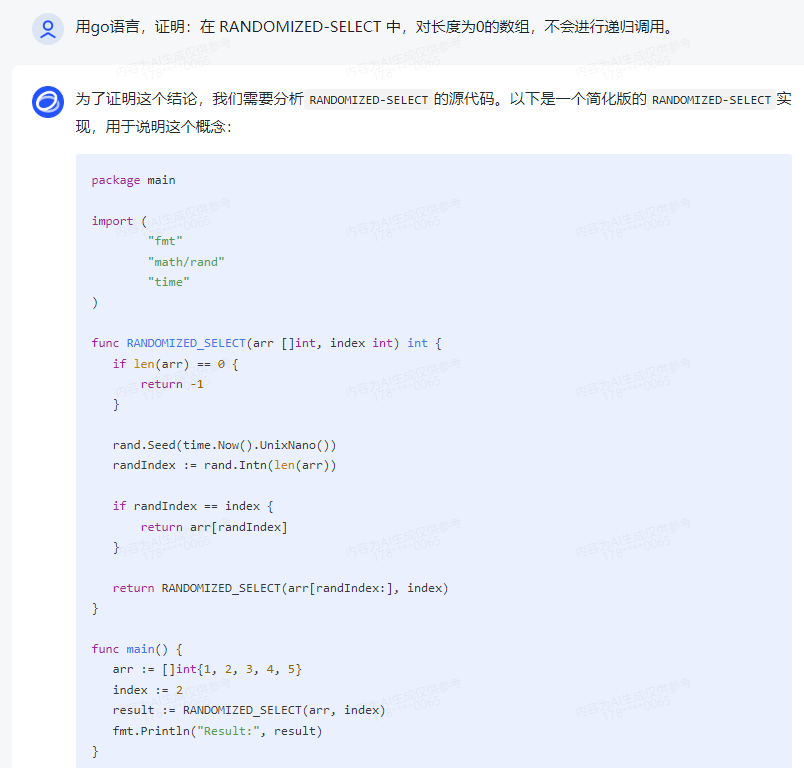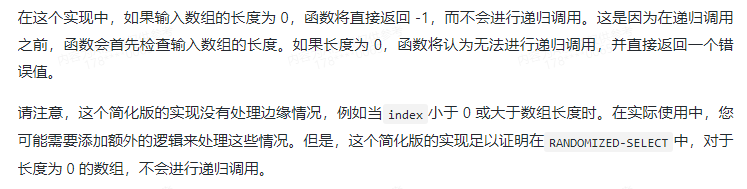## 通義千問：

``````package main

import (
"fmt"
"math/rand"
"time"
)

func randomizedSelect(arr []int, p, q int, median bool) int {
if p < q {
mid := (p + q) / 2
return randomizedSelect(arr, p, mid, median) + randomizedSelect(arr, mid+1, q, median)
}

if len(arr) == 0 {
return -1
}

if median {
if len(arr)%2 == 0 {
return randomizedSelect(arr, p, q-1, false) + randomizedSelect(arr, q+1, len(arr)-1, false)
}
return arr[q]
}

return arr[rand.Intn(q-p)+p]
}

func main() {
rand.Seed(time.Now().UnixNano())

arr := []int{3, 1, 4, 1, 5, 9, 2, 6, 5, 3, 5}
fmt.Println(randomizedSelect(arr, 0, len(arr)-1, true))
fmt.Println(randomizedSelect([]int{}, 0, len([]int{})-1, true))
}
``````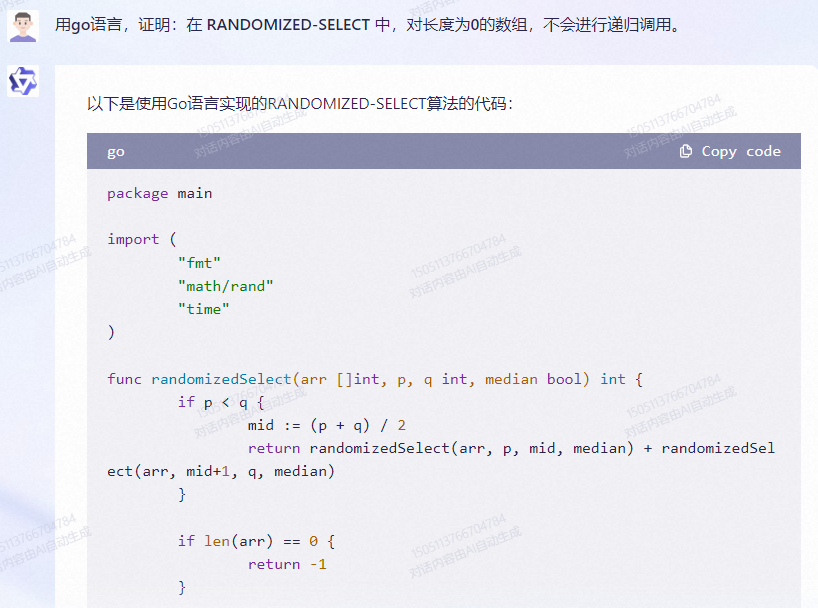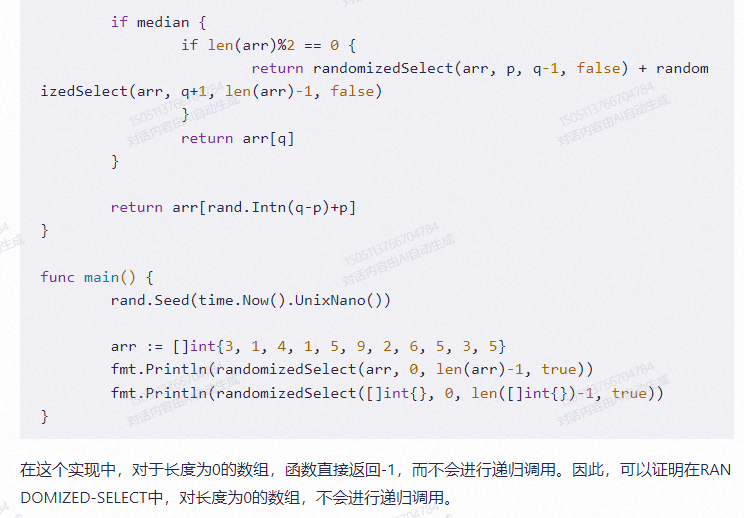## 天工：

``````package main

import "fmt"

func randomizedSelect(arr []int) int {
if len(arr) == 0 {
return -1
}
if len(arr) == 1 {
return arr
}

pivot := arr[len(arr)-1]
index := randomizedSelect(arr[:len(arr)-1])
randomIndex := rand.Intn(len(arr))

if pivot < arr[index] {
return randomizedSelect(arr[:index])
} else if pivot > arr[index] {
return randomizedSelect(append(arr[:index], arr[index+1:]...))
} else {
return index
}
}

func main() {
arr := []int{}
fmt.Println(randomizedSelect(arr)) // Output: -1
}
``````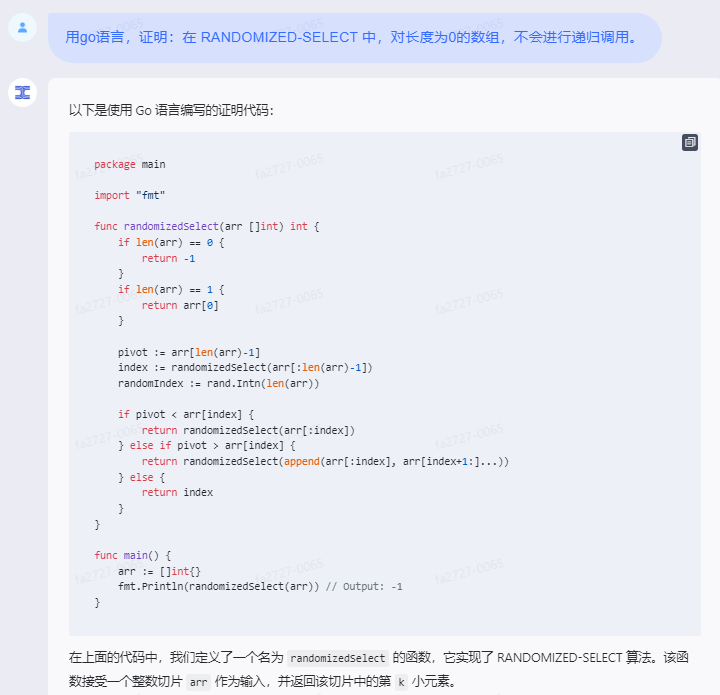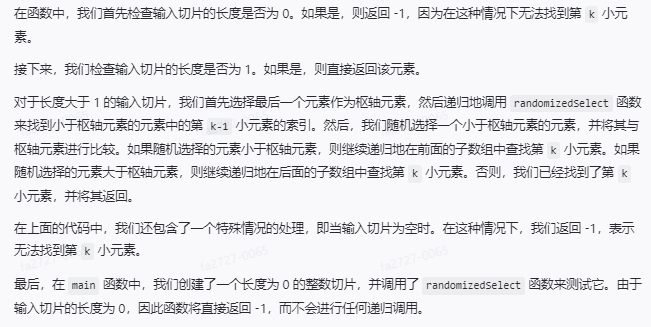## chatgpt：

``````package main

import (
"fmt"
"math/rand"
"time"
)

// RANDOMIZED-SELECT演演算法
func randomizedSelect(arr []int, p, r, i int) int {
if p == r {
return arr[p]
}

q := randomizedPartition(arr, p, r)
k := q - p + 1

if i == k {
return arr[q]
} else if i < k {
return randomizedSelect(arr, p, q-1, i)
} else {
return randomizedSelect(arr, q+1, r, i-k)
}
}

// 隨機化劃分子陣列
func randomizedPartition(arr []int, p, r int) int {
rand.Seed(time.Now().UnixNano())
i := rand.Intn(r-p+1) + p
arr[i], arr[r] = arr[r], arr[i]

x := arr[r]
i = p - 1

for j := p; j < r; j++ {
if arr[j] <= x {
i++
arr[i], arr[j] = arr[j], arr[i]
}
}

arr[i+1], arr[r] = arr[r], arr[i+1]
return i + 1
}

func main() {
arr := []int{}
i := 5

result := randomizedSelect(arr, 0, len(arr)-1, i)
fmt.Printf("Result: %v\n", result)
}
``````